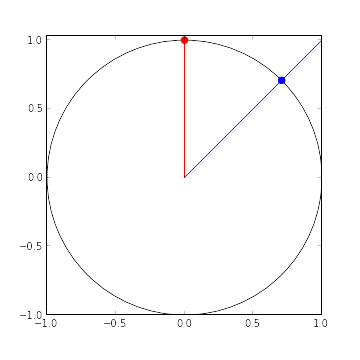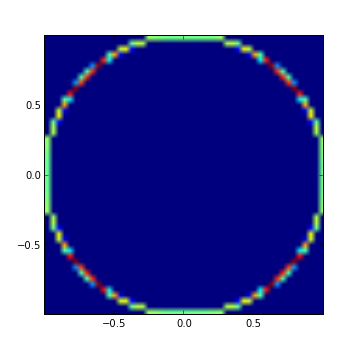# My Most Interesting Interview Problem

I find most short interview problems quite contrived. This post is devoted to the one interview problem that I have found most interesting, due to the simplicity of its statement and the subtlety of its solution.

Consider the following function, intended to generate points uniformly distributed on the unit circle, $x^2 + y^2 = 1$.

import numpy as np
from scipy.stats import uniform

def generate(size=1)
# Generate a point uniformly distributed in the square [-1, 1] x [-1, 1]
x = uniform.rvs(loc=-1, scale=2, size=size)
y = uniform.rvs(loc=-1, scale=2, size=size)

# Normalize by the distance from the origin to get a point on the unit circle
r = np.sqrt(x**2 + y**2)
return np.column_stack([x / r, y / r])

The question asks whether or not this code does indeed generate points uniformly distributed on the unit circle.

At first glance it seems reasonable that it might do so. Upon slightly further reflection, we see that for the results to be uniformly distributed, we must map the square $[-1, 1] \times [-1, 1]$ to the unit circle in a manner that preserves area (in the appropriate sense). From this point of view, we can quickly conclude that the results will not be uniformly (EDIT: this originally read “normally,” which was a typo) distributed.

To see why, consider the following diagram.All of the points in the square on the red line get scaled to the red point on the unit circle. Likewise, all of the points in the square on the blue line get scaled to the blue point on the unit circle. It is quite clear from this diagram that the blue line is longer than the red line. To be precise, the red line has length one, while the blue line has length $\sqrt{2} \approx 1.414.$

Due to this difference in length, the points generated by the function are more likely to be clustered around the four points $(\sqrt{2}, \sqrt{2})$, $(-\sqrt{2}, \sqrt{2})$, $(-\sqrt{2}, -\sqrt{2})$, and $(\sqrt{2}, -\sqrt{2})$ than elsewhere on the circle.

The following diagram shows a heatmap of the locations of one million samples generated by this function.We can see that the samples do indeed cluster around those four points, as shown by the red regions in the heatmap.

Although this problem is fairly simple, unlike many interview problems it reinforces a key breakdown in our intuition about probability theory and naive interpretations of transformations of random variables.

Discuss on Hacker News

Update

Many people have pointed out that this isn’t a great problem for general developer interview, and I agree. This interview was for a data-oriented position which required a strong understanding of probability theory.